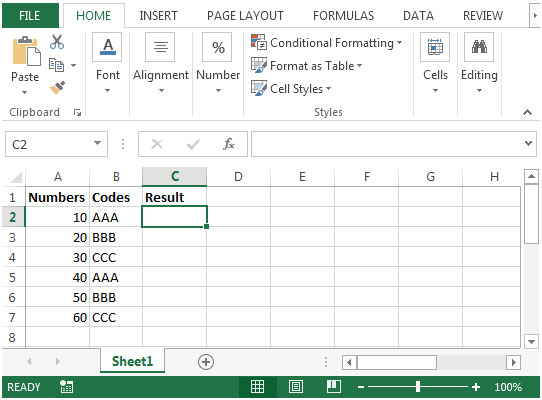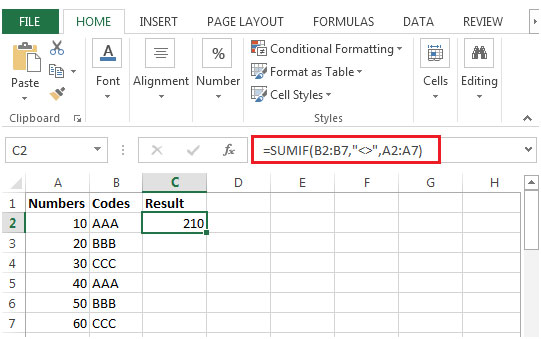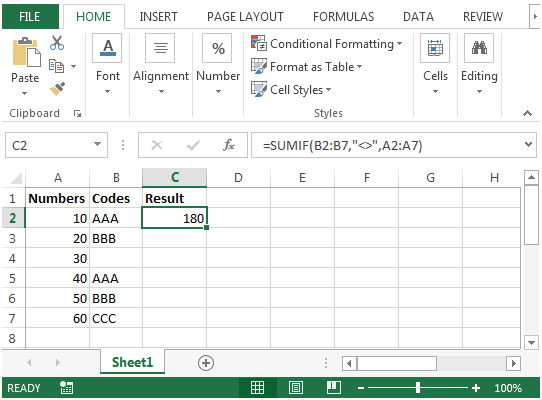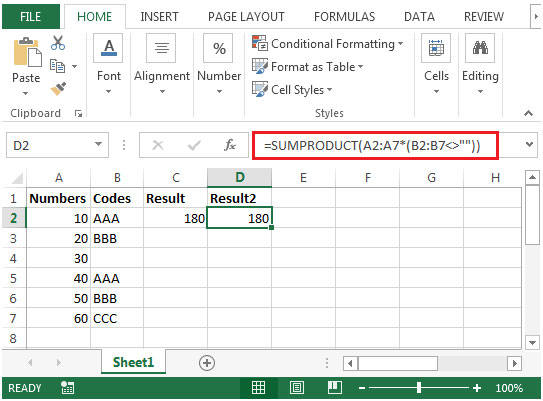# Summing Values Based on the Criteria of a Non-Empty Adjacent Cell

While working on reports, you want a formula that will give you a sum of values based on the criteria of a corresponding non-empty cell.

You can find the output by using SUMIF & SUMPRODUCT functions.
Let us take an example:

We have some numbers in column A & some codes in column B. In column C, we want a formula that will give us the total of numbers in column A provided that the corresponding cell in column B should not be empty. If it is empty then the formula will ignore the value in column A• In cell C2, the formula would be =SUMIF(B2:B7,"<>",A2:A7)• The criterion used is<> (not equal to) to derive the output.
• If we remove any code from column B then the output will be impacted.• We have removed code from cell B4 & the formula has ignored the value in corresponding cell in column A i.e. 30. Hence, we get 180 (210-30) as our output.

Let us see the second method by which we can get the same output. We can use SUMPRODUCT function to obtain the same output.

• The formula in cell D2 would be =SUMPRODUCT(A2:A7*(B2:B7<>""))
• The same result we will get.## Users are saying about us...

1.In some cases we may also want to exclude the “non-empty-but-blank” cells which are the result of a conditional formula retuning the value “” or '.
If this is the case the formula =SUMIF(B2:B7,"",A2:A7) will not exclude these “non-empty-but-blank” cells,
however these cells are excluded by the second formula =SUMPRODUCT(A2:A7*(B2:B7””))

Terms and Conditions of use

The applications/code on this site are distributed as is and without warranties or liability. In no event shall the owner of the copyrights, or the authors of the applications/code be liable for any loss of profit, any problems or any damage resulting from the use or evaluation of the applications/code.